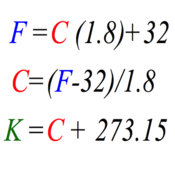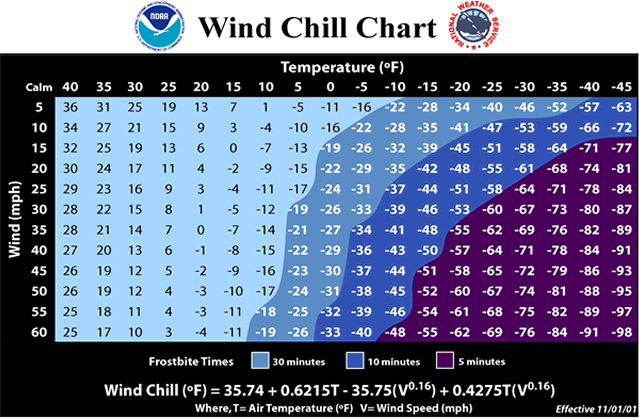Chapter 3-- Temperature

UPDATE AS OCTOBER 10TH -- TEST MOVED TO OCT 18TH Test 2 is OCTOBER 18TH on chapters 3 - 4 and 5 ---- Test 2 is OCTOBER 18

Temperature is one of the most basic elements of weather and climate.

The daily mean temperature is determined by averaging the 24 hourly readings or by adding the maximum and minimum temperatures for a 24-hour period and dividing by two. The daily temperature range is computed by finding the difference between the maximum and minimum temperatures. Other temperature data involving longer periods include the monthly mean temperature (the sum of the daily means for each day of the month divided by the number of days in the month), the annual mean temperature (the average of the twelve monthly temperatures), and the annual temperature range (the difference between the warmest and coldest monthly mean temperatures).
Climate data

The controls of temperature, those factors that cause temperature to vary from place to place, are:

(1) differential heating of land and water
(2) ocean currents
(3) altitude
(4) geographic position
(5) cloud cover
(6) albedo

On maps illustrating the world distribution of temperature, isotherms, lines that connect points of the same temperature, generally trend east and west and show a decrease in temperature poleward. Moreover, the isotherms illustrate a latitudinal shifting of temperatures caused by the seasonal migration of the Sun's vertical rays and also reveal the presence of ocean currents. The north-south migration of isotherms is more pronounced over the continents because the temperatures do not fluctuate as much over water.

Annual temperature range is small near the equator and increases with an increase in latitude. Outside the tropics, annual temperature range also increases with an increase in continentality. For example, Islip, NY has a summer time high of 83 and a winter time high of 38. Miami, Fl: Summertime High: 92 Winter: 77.

The primary control of daily temperature changes is Earth's rotation. However, the magnitude of these changes is variable and influenced by local factors, local weather conditions, or both.

As a consequence of the mechanism by which Earth's atmosphere is heated, the months of the highest and lowest temperatures do not coincide with the periods of maximum and minimum incoming solar radiation. Although the greatest intensity of solar radiation occurs at the time of the summer solstice, in the Northern Hemisphere the months of July and August are generally the warmest of the year. Conversely, in the Northern Hemisphere a minimum of solar energy is received in December at the time of the winter solstice, but January and February are usually colder.Thermometers measure temperature either mechanically or electrically. Most mechanical thermometers are based on the ability of a substance to expand when heated and contract when cooled.

One type of mechanical thermometer, the liquid-in-glass thermometer, includes maximum thermometers, which use liquid mercury, and minimum thermometers, which contain a liquid of low density, such as alcohol. A bimetal strip mechanical thermometer is frequently used in a thermograph, an instrument that continuously records temperature. Electrical thermometers use a thermistor (a thermal resistor) to measure temperature.

Temperature scales use reference points, called fixed points.

Three common temperature scales are

The focus is more on Fahrenheit scale and Celsius scale.

(1) the Fahrenheit scale, which is defined by using the ice point (32°) and steam point (212°)
(2) the Celsius scale, a decimal scale on which the melting point of ice is set at 0 and the boiling point of water at 100°
(3) the Kelvin or absolute scale, where the zero point represents the temperature at which all molecular motion is presumed to cease (called absolute zero), the ice point is set at 273, and the steam point at 373.

Convert Celsius to Kelvin: K = °C + 273
Convert Celsius to Fahrenheit: °F = 1.8(°C) + 32

Convert Fahrenheit to Celsius: = °C(5/9)*(T°F-32)

Example C to F...16 °C = °F , °F = 1.8 x 16°C =28.8 + 32 = 61°F

Example F to C.. °C = 5/9*(61°F-32).. 5/9*29 =16.1°C... 5/9= .55555555Please watch the Below Videos - we will only be converting ---Look at pages 72 and 73 -- Very Important --
Convert Celsius to Fahrenheit: °F = 1.8(°C) + 32
Convert Fahrenheit to Celsius: = °C(5/9)*(T°F-32) or °C = °F - 32/1.8

More conversions

Three common applications of temperature indices are

(1) heating degree days, where each degree of temperature below 65°F is counted as one heating degree day
(2) cooling degree days, which are determined by subtracting 65°F from the daily mean
(3) growing degree days, which are determined from the difference between the daily mean temperature and the base temperature of the crop, the minimum temperature required for it to grow.

Climate heating/Cooling days fo Islip

One familiar use of temperature data relates to human perception of temperature. The heat stress index (or heat index), a commonly used expression of summertime discomfort, links humidity and temperature to determine the thermal comfort of the human body.
Heat Index ChartWindchill, a typical wintertime index, uses both wind and air temperature to calculate the human sensation of temperature.
Wind Chill Chart1. Isotherm- A line of equal temperature. Each of the analysis charts will show isotherms in either a 2,4,5 or 10 degree increment. They are most commonly used at pressure surfaces below 500 millibars and on surface charts. Isotherms are used to find regions with warm air advection and cold air advection as well as short waves, fronts, temperature gradient boundaries, and instability zones.

FOR TEST 2 -- Some basic concepts -- Read the Textbook Chapter 3. TEST MOVED TO OCT 18TH Test 2 is OCTOBER 18TH on chapters 3 - 4 and 5 ---- Test 2 is OCTOBER 18--Chapter 3, 4 and 5.

Need to know how to convert temperature scales

What is Temperature and How do we measure

What is a heating degree day? What is a Cooling Degree Day?

What controls the temperature? How can temperature change?
Temperature scales use reference points, called fixed points.